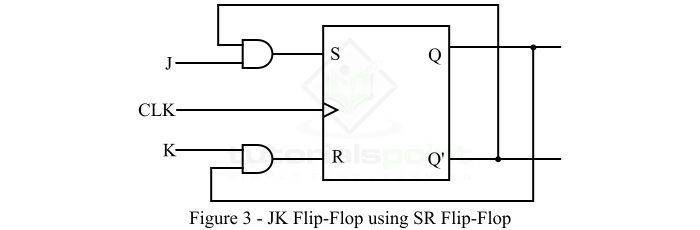# Conversion of SR Flip-Flop to JK Flip-Flop

## What is SR Flip-Flop?

SR flip-flop is a simple 1-bit storage element which has two inputs namely S and R, and two outputs, i.e. Q and Q'. Where, S specifies Set input and R specifies Reset input. The output Q is the normal output and the Q' is the complemented or inverted output. In addition to SR inputs, the SR flip flop also has a clock input which is used for triggering the circuit.

The block diagram of SR flip flop is shown in Figure-1 below.The operation of the SR flip flop can be analyzed using its truth table, which is given below.

Inputs Output
S R Qn+1
00Qn
010
101
11Forbidden

Here, Qn+1 is the next state, and Qn is the present state of the output.

The characteristic equation of the SR flip flop is given by,

$$Q_{n+1}=S+R'Q_{n}$$

## What is JK Flip-Flop?

JK flip flop is also a 1-bit storage device having two inputs similar to SR flip flop, but it has inputs denoted by J and K instead of S and R. It has two outputs viz. Q (normal output) and Q' (inverted output). The clock signal is used for synchronization of the circuit.

The block diagram of the JK flip flop is shown in Figure-2 below.The operation of the JK flip flop can be understood with the help of its truth table which is given below −

Inputs Output
JKQn+1
00Qn
010
101
11Toggle

The characteristic equation of the JK flip flop is given by,

$$Q_{n+1}=JQ_{n}'+K'Q_{n}$$

After discussing about the basics of SR flip flop and JK flip flop. Let us now discuss the conversion of SR flip flop into JK flip flop.

## Conversion of Flip-Flop

We can convert a one type of flip-flop into another type of flip-flop. The conversion process of flip-flops involves the following steps −

• Step 1 − Write the excitation table of the flip flops.

• Step 2 − Simplify the excitation table with the help of Karnaugh Map (K-map).

• Step 3 − Draw the required logic circuit diagram.

Now, let us convert the SR flip-flop into the JK flip-flop.

## Conversion of SR Flip-Flop into JK Flip-Flop

As per the steps of conversion of flip-flops, the conversion process of SR flip-flop into JK flip-flop is described below.

Step 1 − The excitation table for the conversion of SR flip-flop into JK flip-flop is given below −

Inputs Present State of Output Next State of Output Flip-Flop Inputs
JKQnQn+1SRSR
0000 00 0X
01
0011 00 X0
10
0 1 0 0 0 0 0 X
0 1
01100101
10011010
1 0 1 1 0 0 X 0
1 0
11011010
11100101

Step 2 − Simplify the excitation for input expressions by using K-Map −

The K-Map simplification for S is,S = JQn'

The K-map simplification for R is,R = KQn

Step 3 − Finally, draw the logic diagram of JK flip flop by using SR flip flop, which is shown in figure-3 below.In this way, we can convert the SR flip-flop into JK flip-flop.

Updated on: 24-Apr-2023

3K+ Views